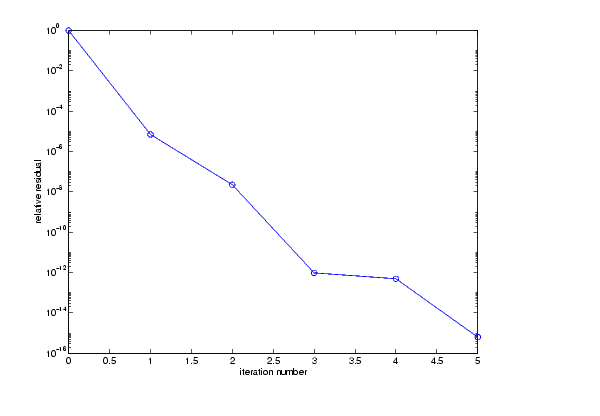MATLAB Function Referencecgs

Conjugate gradients squared method

Syntax

• ```x = cgs(A,b)
cgs(A,b,tol)
cgs(A,b,tol,maxit)
cgs(A,b,tol,maxit,M)
cgs(A,b,tol,maxit,M1,M2)
cgs(A,b,tol,maxit,M1,M2,x0)
[x,flag] = cgs(A,b,...)
[x,flag,relres] = cgs(A,b,...)
[x,flag,relres,iter] = cgs(A,b,...)
[x,flag,relres,iter,resvec] = cgs(A,b,...)
```

Description

```x = cgs(A,b) ``` attempts to solve the system of linear equations `A*x = b` for `x`. The `n`-by-`n` coefficient matrix `A` must be square and should be large and sparse. The column vector `b` must have length `n`. `A` can be a function handle `afun` such that `afun(x)` returns `A*x`. See Function Handles in the MATLAB Programming documentation for more information.

Parameterizing Functions Called by Function Functions, in the MATLAB Mathematics documentation, explains how to provide additional parameters to the function `afun`, as well as the preconditioner function `mfun` described below, if necessary.

If `cgs` converges, a message to that effect is displayed. If `cgs` fails to converge after the maximum number of iterations or halts for any reason, a warning message is printed displaying the relative residual `norm(b-A*x)/norm(b)` and the iteration number at which the method stopped or failed.

```cgs(A,b,tol) ``` specifies the tolerance of the method, `tol`. If `tol` is `[]`, then `cgs` uses the default, `1e-6`.

```cgs(A,b,tol,maxit) ``` specifies the maximum number of iterations, `maxit`. If `maxit` is `[]` then `cgs` uses the default, `min(n,20)`.

```cgs(A,b,tol,maxit,M) and cgs(A,b,tol,maxit,M1,M2) ``` use the preconditioner `M` or `M = M1*M2` and effectively solve the system `inv(M)*A*x = inv(M)*b` for `x`. If `M` is `[]` then `cgs` applies no preconditioner. `M` can be a function handle `mfun` such that `mfun(x)` returns `M\x`.

```cgs(A,b,tol,maxit,M1,M2,x0) ``` specifies the initial guess `x0`. If `x0` is `[]`, then `cgs` uses the default, an all-zero vector.

```[x,flag] = cgs(A,b,...) ``` returns a solution `x` and a flag that describes the convergence of `cgs`.

 Flag Convergence `0` `cgs` converged to the desired tolerance `tol` within `maxit `iterations. `1` `cgs` iterated `maxit` times but did not converge. `2` Preconditioner `M` was ill-conditioned. `3` `cgs` stagnated. (Two consecutive iterates were the same.) `4` One of the scalar quantities calculated during `cgs` became too small or too large to continue computing.

Whenever `flag` is not `0`, the solution `x` returned is that with minimal norm residual computed over all the iterations. No messages are displayed if the `flag` output is specified.

```[x,flag,relres] = cgs(A,b,...) ``` also returns the relative residual `norm(b-A*x)/norm(b)`. If `flag` is `0`, then `relres <= tol`.

```[x,flag,relres,iter] = cgs(A,b,...) ``` also returns the iteration number at which `x` was computed, where `0 <= iter <= maxit`.

```[x,flag,relres,iter,resvec] = cgs(A,b,...) ``` also returns a vector of the residual norms at each iteration, including `norm(b-A*x0)`.

Examples

Example 1.

• ```A = gallery('wilk',21);
b = sum(A,2);
tol = 1e-12;  maxit = 15;
M1 = diag([10:-1:1 1 1:10]);
x = cgs(A,b,tol,maxit,M1);
```

displays the message

• ```cgs converged at iteration 13 to a solution with relative residual
1.3e-016
```

Example 2.

This example replaces the matrix `A` in Example 1 with a handle to a matrix-vector product function `afun`, and the preconditioner `M1` with a handle to a backsolve function `mfun`. The example is contained in an M-file `run_cgs` that

• Calls `cgs` with the function handle `@afun` as its first argument.
• Contains `afun` as a nested function, so that all variables in `run_cgs` are available to `afun` and `myfun`.

The following shows the code for `run_cgs`:

• ```function x1 = run_cgs
n = 21;
A = gallery('wilk',n);
b = sum(A,2);
tol = 1e-12;  maxit = 15;
x1 = cgs(@afun,b,tol,maxit,@mfun);

function y = afun(x)
y = [0; x(1:n-1)] + ...
[((n-1)/2:-1:0)'; (1:(n-1)/2)'].*x + ...
[x(2:n); 0];
end

function y = mfun(r)
y = r ./ [((n-1)/2:-1:1)'; 1; (1:(n-1)/2)'];
end
end
```

When you enter

• ```x1 = run_cgs
```

MATLAB returns

• ```cgs converged at iteration 13 to a solution with relative residual
1.3e-016
```

Example 3.

• ```load west0479
A = west0479
b = sum(A,2)
[x,flag] = cgs(A,b)
```

`flag` is `1` because `cgs` does not converge to the default tolerance `1e-6` within the default 20 iterations.

• ```[L1,U1] = luinc(A,1e-5)
[x1,flag1] = cgs(A,b,1e-6,20,L1,U1)
```

`flag1` is `2` because the upper triangular `U1` has a zero on its diagonal, and `cgs` fails in the first iteration when it tries to solve a system such as `U1*y = r` for `y` with backslash.

• ```[L2,U2] = luinc(A,1e-6)
[x2,flag2,relres2,iter2,resvec2] = cgs(A,b,1e-15,10,L2,U2)
```

`flag2` is `0` because `cgs` converges to the tolerance of `6.344e-16` (the value of `relres2`) at the fifth iteration (the value of `iter2`) when preconditioned by the incomplete LU factorization with a drop tolerance of `1e-6`. `resvec2(1) = norm(b)` and `resvec2(6) = norm(b-A*x2)`. You can follow the progress of `cgs` by plotting the relative residuals at each iteration starting from the initial estimate (iterate number 0) with

• ``````semilogy(0:iter2,resvec2/norm(b),'-o')
```xlabel('iteration number')
ylabel('relative residual')```

See Also

`bicg`, `bicgstab`, `gmres`, `lsqr`, `luinc`, `minres`, `pcg`, `qmr`, `symmlq`

`function_handle` (`@`), `mldivide` (`\`)

References

  Barrett, R., M. Berry, T. F. Chan, et al., Templates for the Solution of Linear Systems: Building Blocks for Iterative Methods, SIAM, Philadelphia, 1994.

  Sonneveld, Peter, "CGS: A fast Lanczos-type solver for nonsymmetric linear systems", SIAM J. Sci. Stat. Comput., January 1989, Vol. 10, No. 1, pp. 36-52.

© 1994-2005 The MathWorks, Inc.